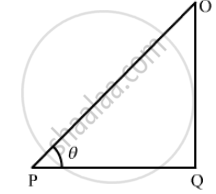Department of Pre-University Education, KarnatakaPUC Karnataka Science Class 11

# A Particle Moves on a Given Straight Line with a Constant Speed ν. at a Certain Time It is at a Point P on Its Straight Line Path. O is a Fixed - Physics

Sum

A particle moves on a given straight line with a constant speed ν. At a certain time it is at a point P on its straight line path. O is a fixed point. Show that $\vec{OP} \times \vec{\nu}$ is independent of the position P.

#### Solution

The particle moves on the straight line XX' at a uniform speed ν.In
$∆ POQ$
OQ = OP sin θ

$\vec{OP} \times \nu = \left( OP \right) \nu \sin \theta \hat n$

$= \nu \left( OP \right) \sin \theta \hat n$

$= \nu\left( OQ \right)\hat n$

This product is always equal to the perpendicular distance from point O. Also, the direction of this product remains constant.
So, irrespective of the the position of the particle, the magnitude and direction of $\vec{OP} \times \vec{\nu}$  remain constant.  $\therefore \vec{OP} \times \vec{\nu}$ is independent of the position P.

Concept: Physics Related to Technology and Society
Is there an error in this question or solution?

#### APPEARS IN

HC Verma Class 11, Class 12 Concepts of Physics Vol. 1
Chapter 2 Physics and Mathematics
Exercise | Q 17 | Page 29

Share